Introduction to SSM Analysis

library(circumplex)

1. Background and Motivation

Circumplex models, scales, and data

Circumplex models are popular within many areas of psychology because they offer a parsimonious account of complex psychological domains, such as emotion and interpersonal functioning. This parsimony is achieved by understanding phenomena in a domain as being a “blend” of two primary dimensions. For instance, circumplex models of emotion typically represent affective phenomena as a blend of valence (pleasantness versus unpleasantness) and arousal (activity versus passivity), whereas circumplex models of interpersonal functioning typically represent interpersonal phenomena as a blend of communion (affiliation versus separation) and agency (dominance versus submissiveness). These models are often depicted as circles around the intersection of the two dimensions (see figure). Any given phenomenon can be located within this circular space through reference to the two underlying dimensions (e.g., anger is a blend of unpleasantness and activity).

Circumplex scales contain multiple subscales that attempt to measure different blends of the two primary dimensions (i.e., different parts of the circle). Although there have historically been circumplex scales with as many as sixteen subscales, it has become most common to use eight subscales: one for each “pole” of the two primary dimensions and one for each “quadrant” that combines the two dimensions. In order for a set of subscales to be considered circumplex, they must exhibit certain properties. Circumplex fit analyses can be used to quantify these properties.

Circumplex data is composed of scores on a set of circumplex scales for one or more participants (e.g., persons or organizations). Such data is usually collected via self-report, informant-report, or observational ratings in order to locate psychological phenomena within the circular space of the circumplex model. For example, a therapist might want to understand the interpersonal problems encountered by an individual patient, a social psychologist might want to understand the emotional experiences of a group of participants during an experiment, and a personality psychologist might want to understand what kind of interpersonal behaviors are associated with a trait (e.g., extraversion).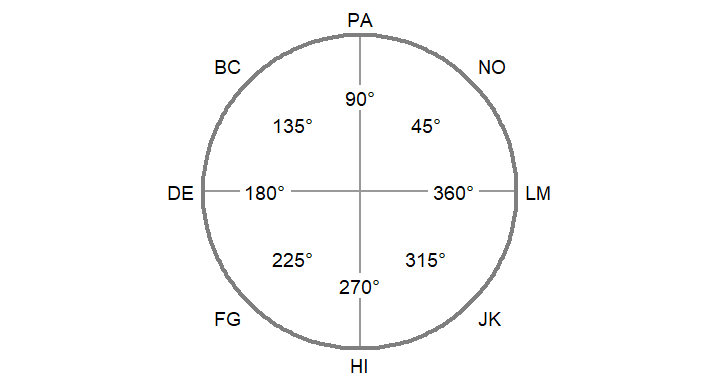The Structural Summary Method

The Structural Summary Method (SSM) is a technique for analyzing circumplex data that offers practical and interpretive benefits over alternative techniques. It consists of fitting a cosine curve to the data, which captures the pattern of correlations among scores associated with a circumplex scale (i.e., mean scores on circumplex scales or correlations between circumplex scales and an external measure). By plotting a set of example scores below, we can gain a visual intuition that a cosine curve makes sense in this case. First, we can examine the scores with a bar chart ignoring the circular relationship among them.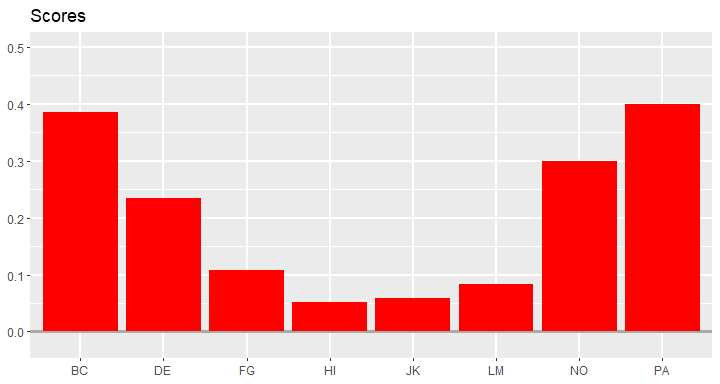Next, we can leverage the fact that these subscales have specific angular displacements in the circumplex model (and that 0 and 360 degrees are the same) to create a path diagram.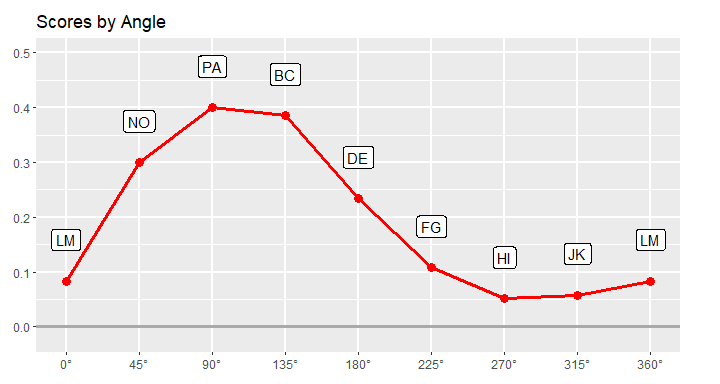This already looks like a cosine curve, and we can finally use the SSM to estimate the parameters of the curve that best fits the observed data. By plotting it alongside the data, we can get a sense of how well the model fits our example data.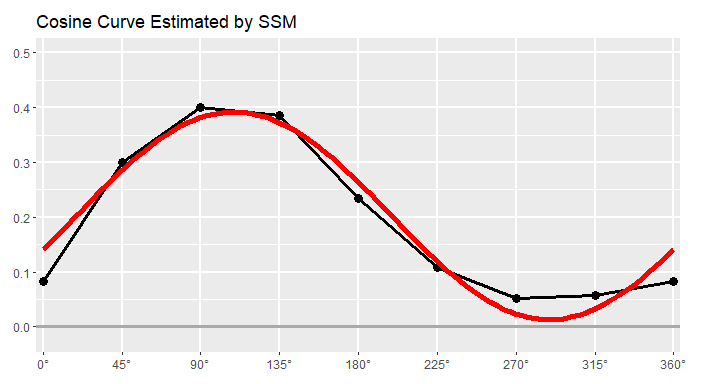Understanding the SSM parameters

The SSM estimates a cosine curve to the data using the following equation: $S_i = e + a \times \cos(\theta_i - d)$ where $$S_i$$ and $$\theta_i$$ are the score and angle on scale $$i$$, respectively, and $$e$$, $$a$$, and $$d$$ are the elevation, amplitude, and displacement parameters, respectively. Before we discuss these parameters, however, we can also estimate the fit of the SSM model. This is essentially how close the cosine curve is to the observed data points. Deviations (in red, below) will lower model fit.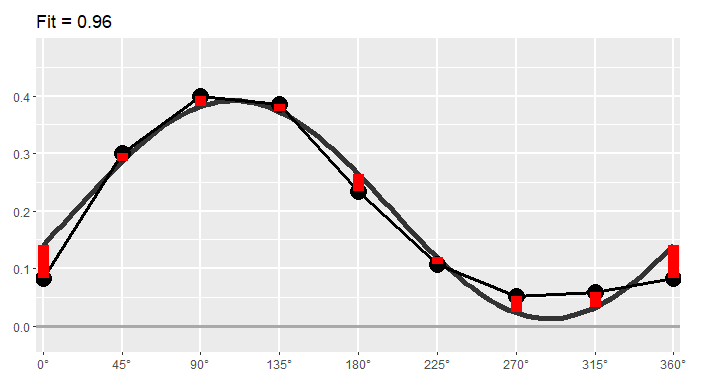If fit is less than 0.70, it is considered “unacceptable” and only the elevation parameter should be interpreted. If fit is between 0.70 and 0.80, it is considered “adequate,” and if it is greater than 0.80, it is considered “good.” Sometimes SSM model fit is called prototypicality or denoted using $$R^2$$.

The first SSM parameter is elevation or $$e$$, which is calculated as the mean of all scores. It is the size of the general factor in the circumplex model and its interpretation varies from scale to scale. For measures of interpersonal problems, it is interpreted as generalized interpersonal distress. When using correlation-based SSM, $$|e|\ge.15$$ is considered “marked” and $$|e|<.15$$ is considered “modest.”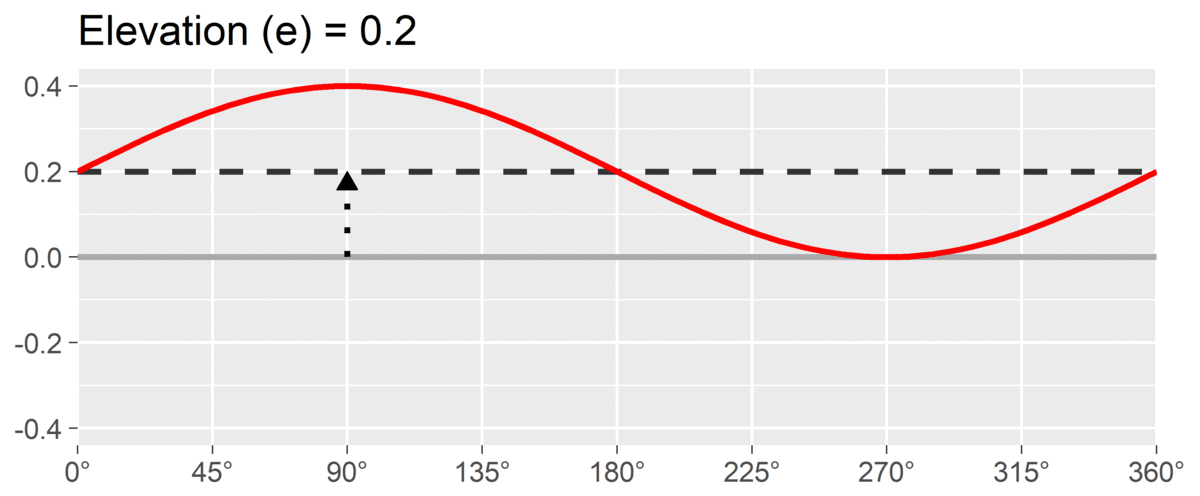The second SSM parameter is amplitude or $$a$$, which is calculated as the difference between the highest point of the curve and the curve’s mean. It is interpreted as the distinctiveness or differentiation of a profile: how much it is peaked versus flat. Similar to elevation, when using correlation-based SSM, $$a\ge.15$$ is considered “marked” and $$a<.15$$ is considered “modest.”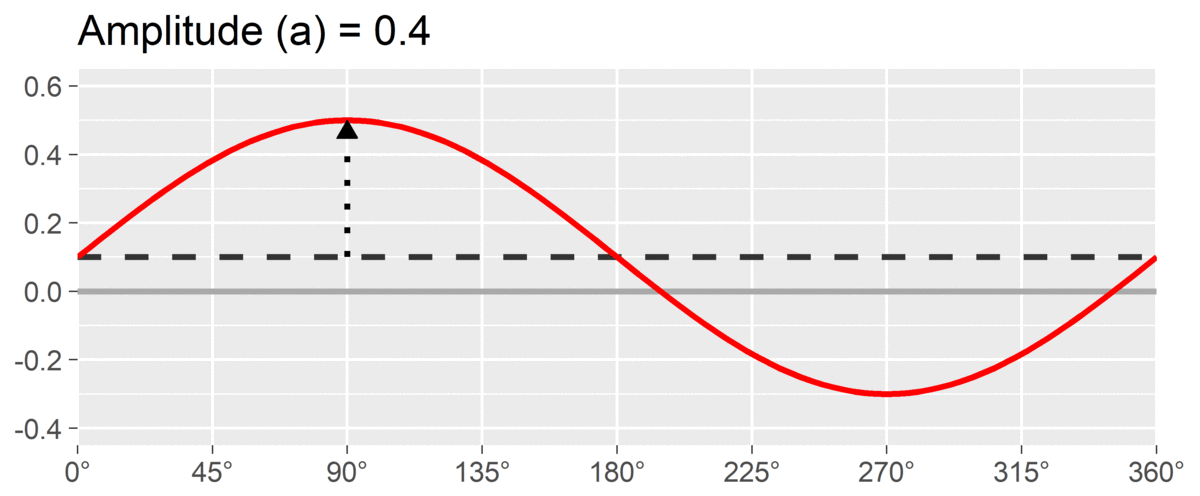The final SSM parameter is displacement or $$d$$, which is calculated as the angle at which the curve reaches its highest point. It is interpreted as the style of the profile. For instance, if $$d=90^\circ$$ and we are using a circumplex scale that defines 90 degrees as “domineering,” then the profile’s style is domineering.